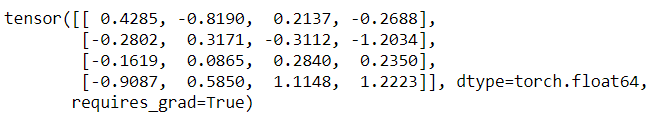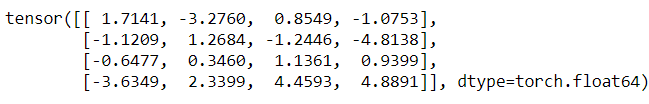### How to Create a Tensor in PyTorch?

PyTorch is a machine learning framework developed  at Facebook's AI Research Lab. It is used in many applications such as computer vision, natural language processing. A PyTorch Tensor is basically same as NumPy array. It is a multidimensional matrix that contains elements of a single data type. A PyTorch Tensor may be one, two or multidimensional. The difference between the NumPy array and PyTorch Tensor is that the PyTorch Tensor can run on the CPU or GPU.Image Source: https://pytorch.org/

#### Creating a PyTorch Tensor

To define a PyTorch Tensor we use torch.tensor() when we use the array or list to create the tensor. Or we can also define a random number tensor using torch.rand(), torch.randn() or torch.randint().

`torch.tensor()  --> to generate a tensor using numpy array or listtorch.rand()    --> to generate a tensor of random numbers from                     a uniform distribution of interval [0,1)torch.randn()   --> to generate a tensor of random numbers from                     a normal distributiontroch.randint() --> to generate a tensor of random integers uniformally`

A PyTorch Tensor can be defined using Python List or numpy array using torch.tensor() constructor.

`torch.tensor([[2.,1.], [3.,4.]])torch.tensor(np.array([[1,2,3],[9,0,2]]))`

Lets have a look on the complete Python program to define 1-D PyTorch Tensor.

`import torchimport numpy as np#define a PyTorch Tensor using Python Lista = torch.tensor([2.,1.,4.,3,4])print(a)# define a PyTorch Tensor using numpy arrayb = torch.tensor(np.array([1,2,3,4,5]))print(b)`

Output:

Lets have a look on the complete Python program to define 2-D PyTorch Tensor.

`import torchimport numpy as np#define a PyTorch Tensor using Python Lista = torch.tensor([[2.,1.,4.], [3.,4.,3],[6,4,3]])print(a)# define a PyTorch Tensor using numpy arrayb = torch.tensor(np.array([[1,2,3],[9,0,2],[2,3,1]]))print(b)`

Output:

Lets create some 1-D PyTorch Tensor of random numbers.

`import torchprint(torch.rand(4))print(torch.randn(4))print(torch.randint(7,(1,4)))`

Output:

Lets generate some 2-D PyTorch Tensor or random numbers.

`import torchprint(torch.rand(3,4))print(torch.randn(3,4))print(torch.randint(7,(3,4)))`

Output:

#### Creating a PyTorch Tensor with requires_grad=True

In deep neural networks, we need to calculate the gradients of the Tensors. When we need to calculate the gradients of the tensors, we can create such tensors providing requires_grad=True.

`# Python 3 program to create a tenor with# requires_grad = Trueimport torchimport numpy as np# take a 4x4 numpy array of random numbersmat = np.random.randn(4,4)# create tnesorx = torch.tensor(mat, requires_grad = True)print(x)`

Output:Fig: PyTorch Tensor with requires_grad=True

We can define a function that performs some operation on the tensor in forward pass of neural network. while backward pass we can calculate the gradients as we put requires_grad=True. See the below Python snippet.

`# define a function out = x.pow(2).sum()# backward passout.backward()# print gradientsprint(x.grad)`

Output:Fig: PyTorch Tensor of gradients

#### Q. How to Access and Modify contents of a PyTorch Tensor?

A.The contents of a PyTorch Tensor can be accessed and modified using the Python indexing and slicing. See the below Python snippet.

`import torcha = torch.tensor([[1,2,3,4],[3,2,5,2]])# access the contentprint(a)print(a)# modify the contentsa = -1print(a)`

Output: